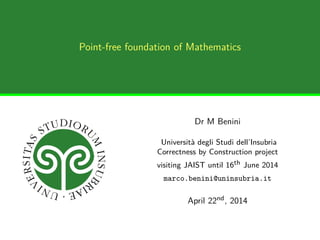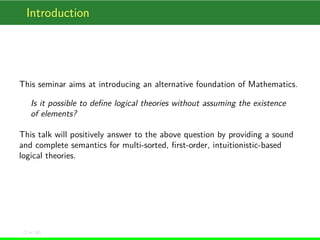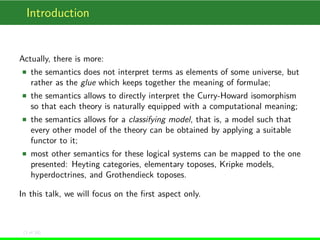Successfully reported this slideshow.

# June 22nd 2014: Seminar at JAIST

0

Share×
1 of 18
1 of 18

# June 22nd 2014: Seminar at JAIST

0

Share

In this talk, logically distributive categories are introduced to provide a sound and complete semantics to multi-sorted, first-order, intuitionistic-based logical theories. The peculiar aspect is that no universe is required to interpret terms, making the semantics really point-free.

In this talk, logically distributive categories are introduced to provide a sound and complete semantics to multi-sorted, first-order, intuitionistic-based logical theories. The peculiar aspect is that no universe is required to interpret terms, making the semantics really point-free.

## More Related Content

### Related Books

Free with a 14 day trial from Scribd

See all

### Related Audiobooks

Free with a 14 day trial from Scribd

See all

### June 22nd 2014: Seminar at JAIST

1. 1. Point-free foundation of Mathematics Dr M Benini Università degli Studi dell’Insubria Correctness by Construction project visiting JAIST until 16th June 2014 marco.benini@uninsubria.it April 22nd, 2014
2. 2. Introduction This seminar aims at introducing an alternative foundation of Mathematics. Is it possible to deﬁne logical theories without assuming the existence of elements? This talk will positively answer to the above question by providing a sound and complete semantics for multi-sorted, ﬁrst-order, intuitionistic-based logical theories. (2 of 18)
3. 3. Introduction Actually, there is more: the semantics does not interpret terms as elements of some universe, but rather as the glue which keeps together the meaning of formulae; the semantics allows to directly interpret the Curry-Howard isomorphism so that each theory is naturally equipped with a computational meaning; the semantics allows for a classifying model, that is, a model such that every other model of the theory can be obtained by applying a suitable functor to it; most other semantics for these logical systems can be mapped to the one presented: Heyting categories, elementary toposes, Kripke models, hyperdoctrines, and Grothendieck toposes. In this talk, we will focus on the ﬁrst aspect only. (3 of 18)
4. 4. Introduction Most of this talk is devoted to introduce a single deﬁnition, logically distributive categories, which identiﬁes the models of our logical systems. These models are suitable categories, equipped with an interpretation of formulae and a number of requirements on their structure. Although the propositional part has already been studied by, e.g., Paul Taylor, the ﬁrst-order extension is novel. (4 of 18)
5. 5. Logically distributive categories Let Σ = 〈S,F,R〉 be a ﬁrst-order signature, with S the set of sort symbols, F the set of function symbols, of the form f : s1 ×···×sn → s0, with si ∈ S for all 0 ≤ i ≤ n, R the set of relation symbols, of the form r : s1 ×···×sn, with si ∈ S for all 1 ≤ i ≤ n. Also, let T be a theory on Σ, i.e., a collection of axioms. A logically distributive category is a pair 〈C,M〉 where C is a category and M a map from formulae on Σ to ObjC, satisfying seven structural conditions, indicated as (C1) to (C7). Informally, objects of C will denote formulae while arrows will denote proofs where the domain is the theorem and the co-domain is the assumption(s). (5 of 18)
6. 6. Logically distributive categories The ﬁrst four conditions allows to interpret propositional intuitionistic logic, as shown in P. Taylor, Practical foundations of mathematics, Cambridge University Press, 1999. (C1) C has ﬁnite products; (C2) C has ﬁnite co-products; (C3) C has exponentiation; (C4) C is distributive, i.e., for every A,B,C ∈ ObjC the arrow ∆ ≡ [1A ×ι1,1A ×ι2] : (A×B)+(A×C) → A×(B +C) has an inverse. Here [_,_] is the co-universal arrow of the (A×B)+(A×C) co-product, 1A is the identity on A, and ι1 : B → B +C, ι2 : C → B +C are the injections of the B +C co-product, _×_ is the product arrow. (6 of 18)
7. 7. Logically distributive categories To express the other conditions, we need additional notation. For every s ∈ S, A formula, and x :s variable, let ΣA(x :s) be the functor from the discrete category of terms of sort s to C, deﬁned by t :s → M(A[t/x]). Also, let C∀x :s.A be the subcategory of C whose objects are the vertexes of the cones on ΣA(x :s) such that each vertex is of the form MB for some formula B with x :s ∈ FVB. The arrows of C∀x :s.A, apart identities, are all the arrows in the category of cones over ΣA(x :s) whose co-domain lies in C∀x :s.A and whose domain is M(∀x :s.A). (7 of 18)
8. 8. Logically distributive categories Dually, C∃x :s.A is the subcategory of C whose objects are the vertexes of the co-cones on ΣA(x :s) such that each vertex is of the form MB for some formula B with x :s ∈ FVB. The arrows of C∃x :s.A, apart identities, are all the arrows in the category of cones over ΣA(x :s) whose domain lies in C∃x :s.A and whose co-domain is M(∃x :s.A). We require that (C5) All the subcategories C∀x :s.A have a terminal object, and all the subcategories C∃x :s.A have an initial object. Evidently, M(∀x :s.A) is the terminal object in C∀x :s.A, and M(∃x :s.A) is the initial object in C∃x :s.A. More important for us, from each object MB in C∀x :s.A there is a unique arrow to M(∀x :s.A), and dually, to each object MB in C∃x :s.A there is a unique arrow from M(∃x :s.A). (8 of 18)
9. 9. Logically distributive categories (C6) We constrain the map M to be as follows: M( ) = 1C, the terminal object of C, M(⊥) = 0C, the initial object of C, M(A∧B) = MA×MB, the binary product in C, M(A∨B) = MA+MB, the binary co-product in C, M(A ⊃ B) = MBMA, the exponential object in C, M(∀x : s.A) = 1C∀x : s.A , the terminal object in C∀x : s.A, M(∃x : s.A) = 0C∃x : s.A , the initial object in C∃x : s.A. Since M is given, the deﬁnition is not circular. But, evidently, it is impredicative. (9 of 18)
10. 10. Logically distributive categories For each variable x :s, A,B formulae with x :s ∈ FVA, it is easy to see that MA×M(∃x :s.B) is an object of C∃x :s.A∧B. Thus, there is a unique arrow δ: M(∃x :s.A∧B) → M(A∧(∃x :s.B)) in C∃x :s.A∧B by (C5). Our last condition is that (C7) the δ arrow above has an inverse in C. (10 of 18)
11. 11. Semantics Given a theory T over a signature Σ and a logically distributive category 〈C,M〉, we interpret each formula on Σ as MA. Given a proof π: Γ T B with Γ = {x1 :A1,...,xn :An}, where assumptions are named x1,...,xn, π will become an arrow x1 :A1,...,xn :An.π:B : A1 ×···×An → B . To lighten notation, the context will be written as x, and A ≡ A1 ×···×An. (11 of 18)
12. 12. Semantics A model for T is a logically distributive category together with a map MAx from T to ObjC such that each axiom A is mapped in an arrow a: 1C → MA. Assuming the standard rules of natural deduction by Prawitz, we inductively interpret proofs as follows: a proof by assumption becomes a projection from the context; a proof by axiom a:B becomes the universal arrow from the context to the terminal object composed by the arrow given by MAx; conjunction eliminations become the projections of the binary product, while conjunction introduction becomes the universal arrow; disjunction elimination become the injections of the binary co-product, while disjunction introduction is reduced to the co-universal arrow; false elimination and truth introduction become the co-universal arrow of the initial object and the universal arrow of the terminal object, respectively; (12 of 18)
13. 13. Semantics universal elimination becomes the projection M(∀x :s.C) → M(A[t/x]) in the unique cone over ΣC (x :s) having M(∀x :s.C) as vertex; universal introduction becomes the universal arrow to the terminal object in the C∀x :s.C subcategory; existential introduction becomes the injection M(A[t/x]) → M(∃x :s.C) in the unique co-cone over ΣC (x :s) having M(∃x :s.C) as vertex; existential elimination becomes the co-universal arrow from the initial object of C∃x :s.A∧C . Actually, the precise deﬁnition is a bit more complex, to take into account the context. Also, some additional properties of the existential and universal subcategories are needed. But this is just technique. . . (13 of 18)
14. 14. Semantics A formula A is valid in the model 〈C,M,MAx〉 when there exists an arrow 1C → MA. A formula A is a logical consequence of B1,...,Bn in the model when there exists an arrow M(B1 ∧...∧Bn) → MA in C. A formula A is a logical consequence of B1,...,Bn when it is so in any model for the theory. (14 of 18)
15. 15. Soundness and completeness Theorem 1 A formula A is a logical consequence of B1,...,Bn in the theory T if and only if there is a proof of A from the hypotheses B1,...,Bn. The proof is long and complex: it can be found in M. Benini, Intuitionistic First-Order Logic: Categorical Semantics via the Curry-Howard Isomorphism, http://arxiv.org/abs/1307.0108, 2013. As a side eﬀect of the completeness proof, it follows that the syntactic category forms a classifying model with respect to the class of functors preserving the logically distributive structure. (15 of 18)
16. 16. The role of terms How terms get interpreted? Variables are used to identify the required subcategories C∀x : s.A and C∃x : s.A; variables are also used to construct the substitution functor ΣA(x :s); all terms contribute to the substitution process, which induces the structure used by the semantics. Thus, it is really the substitution process, formalised in the ΣA(x :s) functors, that matters: terms are just the glue that enable us to construct the C∀x : s.A and C∃x : s.A subcategories. It is clear the topological inspiration of the whole construction. In particular, it is evident that terms are not interpreted in some universe, and their role is limited to link together formulae in subcategories that control how quantiﬁers are interpreted. (16 of 18)
17. 17. Inconsistent theories A theory T is inconsistent when it allows to derive falsity. However, in our semantics, T has a model as well. A closer look to each model of T reveals that they are categorically “trivial” in the sense that the initial and the terminal objects are isomorphic. This provides a way to show that a theory is consistent. However, this is not ultimately easier or diﬀerent than ﬁnding an internal contradiction in the theory. Actually, it does make sense in a purely computational view that an inconsistent theory has a model: it means that, although the speciﬁcation of a program is ultimately wrong, there are pieces of code which are perfectly sound. (17 of 18)
18. 18. Conclusion Much more than this has been done on logically distributive categories. But, still, I am in the beginning of the exploration of this foundation setting. So, any question, comment, suggestion is welcome! Questions? (18 of 18)